# Why does a parallelogram with two diagonals end up making four triangles of the same area?

• Module 2 Week 1 Day 4 Challenge Explanation Part 2

Can you please explain why a parallelogram with two diagonals ends up making four triangles of the same area?

• @CoherentMangoThis is a really interesting and surprising characteristic of parallelograms, that the four triangles formed from the intersection of their diagonals have the same area! I'm glad you asked about it.

Many facts go into this concept:

## Fact 1: Diagonals of a parallelogram bisect each other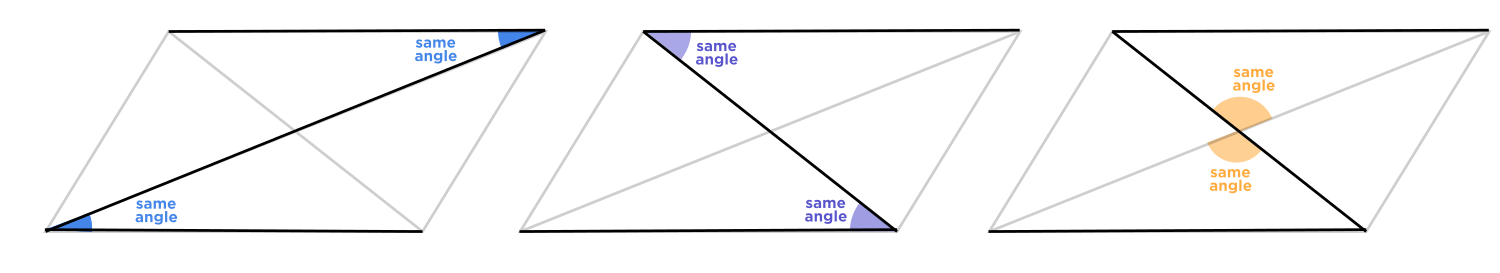Using the parallel lines of the parallelogram, we get the above congruent angles. To give the technical term for these congruent angles, the $$\textcolor{blue}{\text{ blue }}$$ and $$\textcolor{purple}{\text{ purple }}$$ ones are called "alternate interior angles," and the $$\textcolor{orange}{\text{yellow}}$$ ones are called "vertical angles."These two triangles have the same angles, so they are congruent, which means that the $$\textcolor{red}{\text{ red }}$$ side and $$\textcolor{green}{\text{ green }}$$ sides are the same length. So, the diagonals of a parallelogram cut each other in half (bisect each other)!

In the same way, the other two diagonal segments are also the same length.## Fact 2: Each of the three sides of a triangle can be thought of a base

There are three ways of thinking of a base and height for a given triangle. This is because there are three sides of a triangle! In the diagram below, I have drawn the three different heights for the $$\textcolor{purple}{\text{ purple }}$$ triangle, as well as the three different heights for the $$\textcolor{orange}{\text{ yellow }}$$ triangle. The heights are color-coded with their matching bases, so the $$\textcolor{red}{\text{ red }}$$ base goes with the $$\textcolor{red}{\text{ red }}$$ altitude, the $$\textcolor{green}{\text{ green }}$$ base goes with the $$\textcolor{green}{\text{ green }}$$ altitude, and the $$\textcolor{purple}{\text{ purple }}$$ base goes with the $$\textcolor{purple}{\text{ purple }}$$ altitude.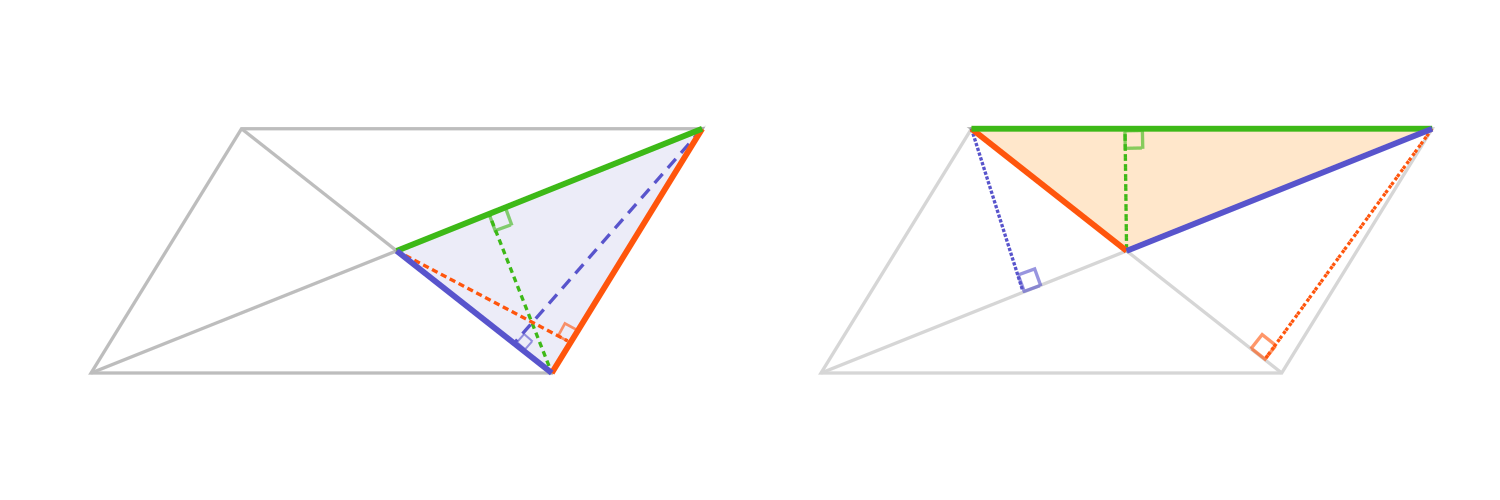An altitude must be perpendicular $$\perp$$ to the base. That means an altitude might have to lie outside the triangle, which is true for obtuse triangles.

Now, looking at the triangles one pair at a time, we see that they all have the same base and height.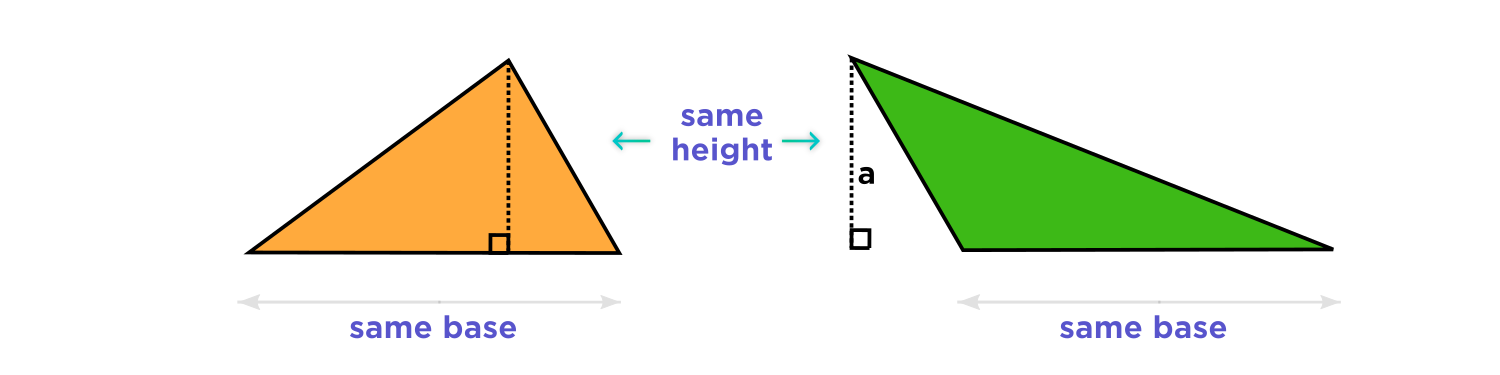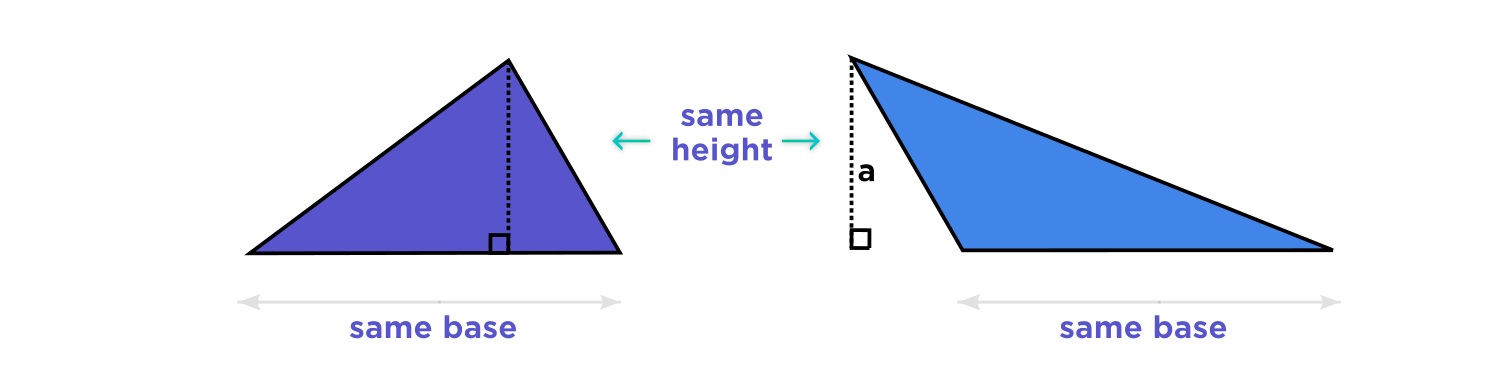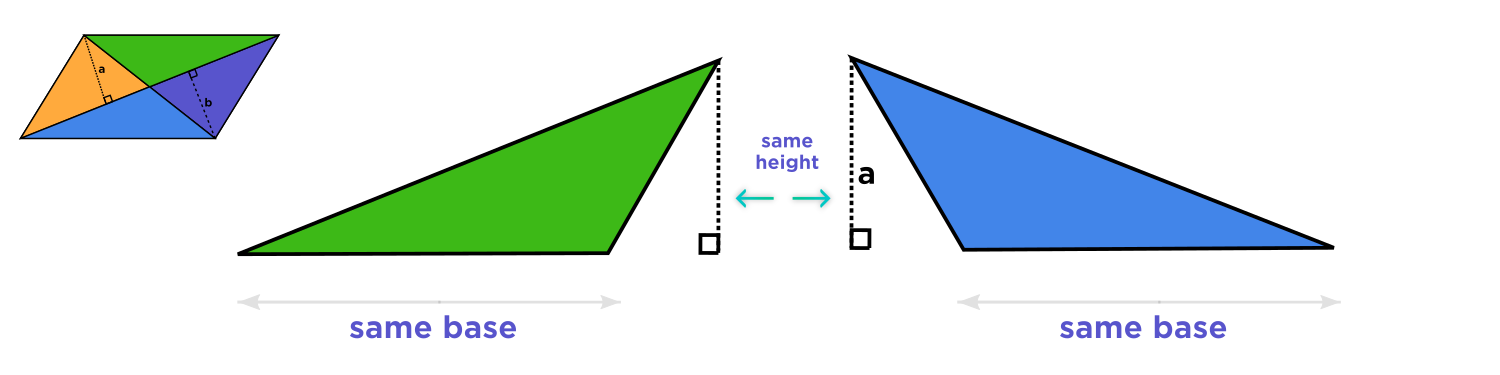Thus the four triangles created by drawing diagonals of any parallelogram each have the same area!

## Even if the bases aren't the same length, you can apply this idea to other triangles

You can generalize this idea in order to find the ratio of areas of triangles which have a common vertex and common base.

In the picture below, the two $$\textcolor{purple}{\text{ purple }}$$ triangles have the same altitude, which is the perpendicular $$\perp$$ line from the top vertex to the base line.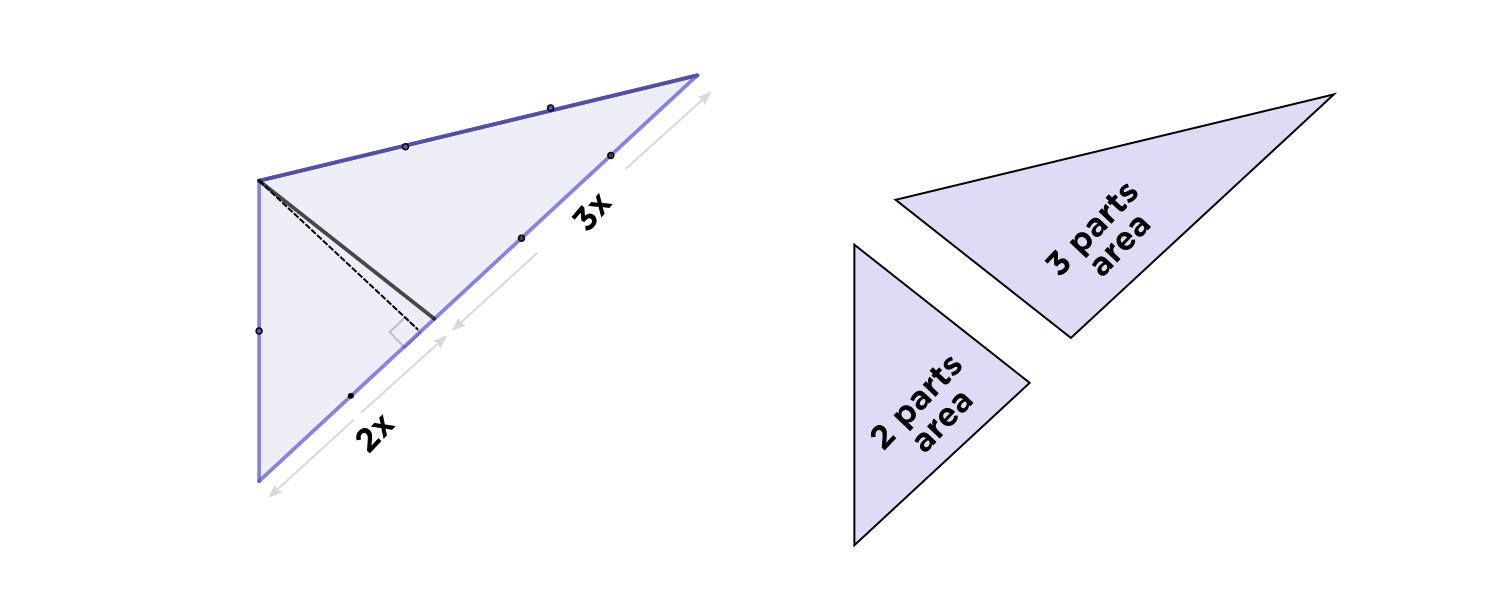Thus to find their relative areas, just look at the relative lengths of their bases. In this example, one base is $$\frac{3}{2} \times$$ the other, and the ratio of their areas is $$2 : 3.$$